GMAT  >   Test: Word Problems - 2

# Test: Word Problems - 2 - GMAT

Test Description

## 15 Questions MCQ Test - Test: Word Problems - 2

Test: Word Problems - 2 for GMAT 2023 is part of GMAT preparation. The Test: Word Problems - 2 questions and answers have been prepared according to the GMAT exam syllabus.The Test: Word Problems - 2 MCQs are made for GMAT 2023 Exam. Find important definitions, questions, notes, meanings, examples, exercises, MCQs and online tests for Test: Word Problems - 2 below.
Solutions of Test: Word Problems - 2 questions in English are available as part of our course for GMAT & Test: Word Problems - 2 solutions in Hindi for GMAT course. Download more important topics, notes, lectures and mock test series for GMAT Exam by signing up for free. Attempt Test: Word Problems - 2 | 15 questions in 30 minutes | Mock test for GMAT preparation | Free important questions MCQ to study for GMAT Exam | Download free PDF with solutions
 1 Crore+ students have signed up on EduRev. Have you?
Test: Word Problems - 2 - Question 1

### Billy has an unlimited supply of the following coins: pennies (1¢), nickels (5¢), dimes (10¢), quarters (25¢), and half-dollars (50¢). On Monday, Billy bought one candy for less than a dollar and paid for it with exactly four coins (i.e., he received no change). On Tuesday, he bought two of the same candy and again paid with exactly four coins. On Wednesday, he bought three of the candies, on Thursday four of the candies, and on Friday five of the candies; each day he was able to pay with exactly four coins. Which of the following could be the price of one candy in cents?

Detailed Solution for Test: Word Problems - 2 - Question 1

We can back solve this quest ion by using the answer choices. Let’s first check to make sure that each of the 5 possible prices for one candy can be paid using exactly 4 coins:
8 = 5+1+1+1
13 = 10+1+1+1
40 = 10+10+10+10
53 = 50+1+1+1
66 = 50+10+5+1
So far we can’t make any eliminations. Now let’s check two pieces of candy:
16 = 5 + 5 + 5 + 1
26 = 10 + 10 + 5 + 1
80 = 25 + 25 + 25 + 5
106 = 50 + 50 + 5 + 1
132 = 50 + 50 + 25 + 5 + 1 + 1
We can eliminate answer choice E here. Now three pieces of candy: 24 = 10 + 10 + 1 + 1 + 1 + 1
39 = 25 + 10 + 1 + 1 + 1 + 1
120 = 50 + 50 + 10 + 10
159 = 50 + 50 + 50 + 5 + 1 + 1 + 1 + 1.
We can eliminate answer choices A, B and D.
Notice that at a price of 40¢, Billy can buy four and five candies with exactly 4 coins as well: 160 = 50 + 50 + 50 + 10
200 = 50 + 50 + 50 + 50
This problem could also have been solved using divisibilit y and remainders. Notice that all o f the coins are mult iples of 5 except pennies. In order to be able to pay for a certain number of candies with exactly four coins, the total price of the candies cannot be a value that can be expressed as 5x + 4, where x is a posit ive integer. In other words, the total price cannot be a number that has a remainder of 4 when divided by 5. Why? The remainder of 4 would alone require 4 pennies.
We can look at the answer choices now just focusing on the remainder when each price and its multiples are divided by 5: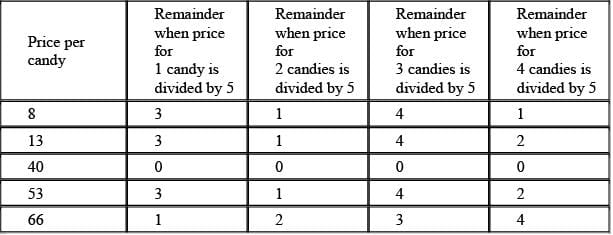The only price for which none of its multiples have a remainder of 4 when divided by 5 is 40¢.
Notice that not having a remainder of 4 does not guarantee that exactly four coins can be used; however, having a remainder of 4 does guarantee that exactly for coins cannot be used!

Test: Word Problems - 2 - Question 2

### A certain violet paint contains 30 percent blue pigment and 70 percent red pigment by weight. A certain green paint contains 50 percent blue pigment and 50 percent yellow pigment. When these paints are mixed to produce a brown paint, the brown paint contains 40 percent blue pigment. If the brown paint weighs 10 grams, then the red pigment contributes how many grams of that weight?

Detailed Solution for Test: Word Problems - 2 - Question 2

From the question we know that 40 percent of the violet/green mix is blue pigment. We also know that 30 percent of the violet paint and 50 percent of the green paint is blue pigment. Since the blue pigment in the violet/green mix is the same blue pigment in the original violet and green paints, we can construct the following equation:
.3v + .5g = .4(v + g)
.3v + .5g = .4v + .4g
.1g = .1v
g = v
Therefore, the amount of violet paint is equal to the amount of green paint in the brown mixture, each contributing 50 percent of the total. Since the red pigment represents 70 percent of the weight of the vio let paint, it must account for 70 percent of 50 percent of the weight of the brown mix. This represents (.7)(.5) = .35, or 35% of the total weight of the brown mix. Since we have 10 grams of the brown paint, the red pigment must account for (.35)(10) = 3.5 grams of the brown paint.
There is an alternative way to come up with the conclusion that there must be equal amounts of green and violet paints in the mix. Since there is blue paint in both the violet and green paints, when we combine the two paints, the percentage of blue paint in the mix will be a weighted average of the percentages of blue in the violet paint and the percentage of blue in the green paint. For example, if there is twice as much violet as green in the brown mix, the percentage of blue in the violet will get double weighted. From looking at the numbers, however, 40% is exactly the simple average of the 30% blue in violet and the 50% blue in green. This means that there must be an equal amount of both paints in the mix.
Since there are equal amounts of violet and green paint in the 10 grams of brown mixture, there must be 5 grams of each. The violet paint is 70% red, so there must be (.7)(5) = 3.5 grams of red paint in the mix.

Test: Word Problems - 2 - Question 3

### The workforce of a certain company comprised exactly 10,500 employees after a four-year period during which it increased every year. During this four-year period, the ratio of the number of workers from one year to the next was always an integer. The ratio of the number of workers after the fourth year to the number of workers after the second year is 6 to 1. The ratio of the number of workers after the third year to the number of workers after the first year is 14 to 1. The ratio of the number of workers after the third year to the number of workers before the four-year period began is 70 to 1. How many employees did the company have after the first year?

Detailed Solution for Test: Word Problems - 2 - Question 3

This quest ion requires us to untangle a series of ratios among the numbers of workers in the various years in order to find the number of workers after the first year. We can solve this problem by setting up a grid to keep track of the information: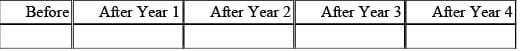We are told initially that after the four-year period, the company has 10,500 employees: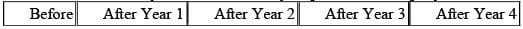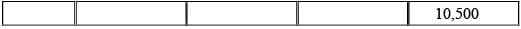We are then told that the ratio of the number of workers after the fourth year to the number of workers after the second year is 6 to 1. This implies that the number of workers after the fourth year is six times greater than that after the second year. Thus the number of workers after the second year must be 10,500/6 = 1,750: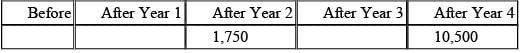We are then told that the ratio of the number of workers after the third year to the number after the first year is 14 to 1. We can incorporate this into the chart: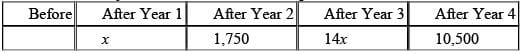Now we are told that the ratio of the number of workers after the third year to that before the period began is 70 to 1. We can incorporate this into the chart as well: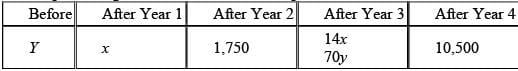From the chart we can see that 14x = 70y. Thus x = 5y: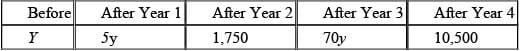Since the ratio between consecut ive years is always an integer and since after three years the number of workers is 70 times greater, we know that the series of ratios for the first three years must include a 2, a 5, and a 7 (because 2 x 5 x 7 = 70). But this fact by itself does not tell us the order of the ratios. In other words, is it 2 - 5 - 7 or 7 - 2 - 5 or 5 - 2 - 7, etc? We do know, however, that the factor of 5 is accounted for in the first year. So we need to know whether the number of workers in the second year is twice as many or seven times as many as in the first year.
Recall that the number of workers after the fourth year is six times greater than that after the second year. This implies that the ratios for the third and fourth years must be 2 and 3 or 3 and 2. This in turn implies that the ratio of 7 to 1 must be between the first and second years. So 1,750 is 7 times greater than the number of workers after the first year. Thus, 1,750/7 = 250.
Alternat ively, since the quest ion states that the ratio between any two years is always an integer, we know that 1,750 must be a multiple of the number of workers after the first year. Since only 70 and 250 are factors of 1750, we know the answer must be either choice B or choice C. If we assume that the number of workers after the first year is 70, however, we can see that this must cannot work. The number of workers always increases from year to year, but if 70 is the number of workers after the first year and if the number of workers after the third year is 14 times greater than that, the number of workers after the third year would be 14 x 70 = 980, which is less than the number of workers after the second year. So choice B is eliminated and the answer must be choice C.

Test: Word Problems - 2 - Question 4

A certain farm has a group of sheep, some of which are rams (males) and the rest ewes (females). The ratio of rams to ewes on the farm is 4 to 5. The sheep are divided into three pens, each of which contains the same number of sheep. If the ratio of rams to ewes in the first pen is 4 to 11, and if the ratio of rams to ewes in the second pen is the same as that of rams to ewes in the third, which of the following is the ratio of rams to ewes in the third pen?

Detailed Solution for Test: Word Problems - 2 - Question 4

It is important to remember that if the ratio of one group to another is x:y, the total number of objects in the two groups together must be a mult iple of x + y. So since the ratio of rams to ewes on the farm is 4 to 5, the total number of sheep must be a mult iple of 9 (4 parts plus 5 parts). And since the ratio of rams to ewes in the first pen is 4 to 11, the total number of sheep in the first pen must be a mult iple of 15 (4 parts plus 11 parts). Since the number of sheep in each pen is the same, the total number of sheep must be a mult iple of both 9 and 15.

If we assume that the total number of sheep is 45 (the lowest common mult iple of 9 and 15), the number of rams is 20 and the number of ewes is 25 (ratio 4:5).
45/3 = 15, so there are 15 sheep in each pen. Therefore, there are 4 rams and 11 ewes in the first pen (ratio 4:11). This leaves 20 - 4 = 16 rams and 25 - 11 = 14 ewes in the other two pens. Since the second and third pens have the same ratio of rams to ewes, they must have 16/2 = 8 rams and 14/2 = 7 ewes each, for a ratio of 8:7 or 8/7.
Alternatively, we can answer the question algebraically.
Since the ratio of rams to ewes in the first pen is 4:11, let the number of rams in the first pen be 4x and the number of ewes be 11x. Let r be the number of rams in the second pen and let e be the number of ewes in the second pen. Since the number of sheep in each pen is the same, we can construct the following equat ion: 4x + 11x = r + e, or 15x = r + e.
Since the number of sheep in each pen is the same, we know that the number of rams in the second and third pens together is 2r and the number of ewes in the second and third pens together is 2e. Therefore, the total number of rams is 4x + 2r. The total number of ewes is 11x + 2e. Since the overall rat io of rams to ewes on t he farm is 4:5, we can construct and simplify the following equation: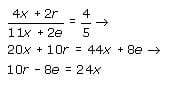We can find the ratio of r to e by setting the equat ions we have equal to each other. First, though, we must multiply each one by coefficients to make them equal the same value: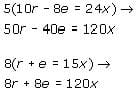Since both equations now equal 120x, we can set them equal to each other and simplify: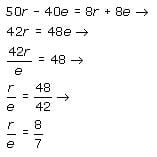Test: Word Problems - 2 - Question 5

The price of a bushel of corn is currently \$3.20, and the price of a peck of wheat is \$5.80. The price of corn is increasing at a constant rate of 5x cents per day while the price of wheat is decreasing at a constant rate of (x√2 – x) cents per day. What is the approximate price when a bushel of corn costs the same amount as a peck of wheat?

Detailed Solution for Test: Word Problems - 2 - Question 5

We can find a ratio between the rates of increase and decrease for the corn and wheat: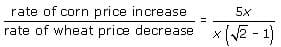To get rid of the radical sign in the denominator, we can multiply top and bottom by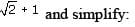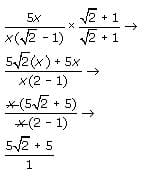This ratio indicates that for every cent that the price of wheat decreases, the price of corn increases by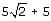cents. So if the price of wheat decreases by x cents, the price of corn will increase by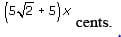Since the difference in price between a peck of wheat and a bushel of corn is current ly \$2.60 or 260 cents, the amount by which the price of corn increases plus the amount by which the price of wheat decreases must equal 260 cents. We can express this as an equation:
Amount Corn Increases + Amount Wheat Decreases = 260 We can then rewrite this word equation using variables. Let c be the decrease in the price of wheat in cents: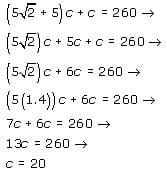Notice that the radical 2 was replaced with its approximate numerical value of 1.4 because the quest ion asks for the approximate price. We need not be exact in this particular instance.
If c = 20, we know that the price of a peck of wheat had decreased by 20 cents when it reached the same level as the increased price of a bushel o f corn. Since the original price of a peck of wheat was \$5.80, its decreased price is \$5.80 - \$0.20 = \$5.60. (By the same token, since c = 20, the price of a bushel of corn had increased by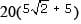cents when it reached the same level as the decreased price of a peck of wheat. This is equivalent to an increase of approximately 240 cents. Thus the increased price of a bushel of corn = \$3.20 + \$2.40 = \$5.60.)

Test: Word Problems - 2 - Question 6

A computer chip manufacturer expects the ratio of the number of defective chips to the total number of chips in all future shipments to equal the corresponding ratio for shipments S1, S2, S3 and S4 combined, as shown in the following table. What is the expected number of defective chips in a shipment of 60,000 chips?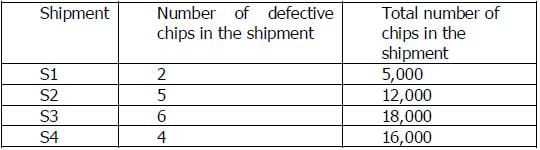Detailed Solution for Test: Word Problems - 2 - Question 6

(2+5+6+4)/(5000+12000+18000+16000)*60000 = 20

Test: Word Problems - 2 - Question 7

A certain library assesses fines for overdue books as follows. On the first day that a book is overdue, the total fine is \$0.10. For each additional day that the book is overdue the total fine is either increased by \$0.30 or double, whichever results in the lesser amount. What is the total fine for a book on the fourth day it is
overdue?

Detailed Solution for Test: Word Problems - 2 - Question 7

The fine for one day: \$0.1 The fine two days: \$0.2, as it is less than \$0.1+\$0.3 The fine for three days: \$0.4, as it is less than \$0.2+\$0.3 The fine for four days: \$0.4+\$0.3=\$0.7, as it is less than \$0.4*2

Test: Word Problems - 2 - Question 8

When a certain tree was first planted, it was 4 feet tall, and the height of the tree increased by a constant amount each year for the next 6 years. At the end of the 6th year, the tree was 1/5 taller than it was at the end of 4th year. By how many feet did the height of the tree increase each year?

Detailed Solution for Test: Word Problems - 2 - Question 8

Let x be the height of the tree increase each year, then: [4+6x-(4+4x)]/(4+4x) = 1/5 10x = 4+ 4x x= 2/3

Test: Word Problems - 2 - Question 9

A certain company plans to sell Product X for p dollars per unit, where p is randomly chosen from all possible positive values not greater than 100. The monthly manufacturing cost for Product X (in thousands of dollars) is 12 - p, and the projected monthly revenue from Product X (in thousands of dollars) is p(6 - p). If the projected revenue is realized, what is the probability that the company will NOT see a profit on sales of Product X in the first month of sales?

Detailed Solution for Test: Word Problems - 2 - Question 9

In order to realize a profit, the company's revenue must be higher than the company's costs. We can express this as an inequality using the information from the question: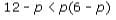If we distribute and move all terms to one side, we get: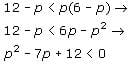We can factor this result: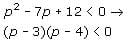When the value of p makes this inequality true, we know we will have a profit. When the value of p does NOT make the inequality true, we will not hav e a profit. When p equals 3 or 4, the product is zero. So the values of p that will make the inequality true (i.e., will yield a negative product) must be either greater than 4, less than 3, or between 3 and 4. To determine which is the case, we can test a sample value from each interval.
If we try p = 5, we get: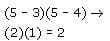Since 2 is positive, we know that values of p greater than 4 will not make the inequality true and thus will not yield a profit.
If we try p = 2, we get: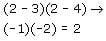Since 2 is positive, we know that the values of p less than 3 will not make the inequality true and thus will not yield a profit.
If we try p = 3.5, we get: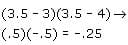Since -.25 is negative, we know that values between 3 and 4 will make the inequality true and will thus yield a profit. Since p can be any positive value less than 100 (we cannot have a negative price or a price of zero dollars), there are 100 possible intervals between consecutive integer values of p. The interval 3 < p < 4 is just one. Therefore, the probability that the comp any will realize a profit is 1/100 and the probability that it will NOT realize a profit is 1 - 1/100 or 99/100.

Test: Word Problems - 2 - Question 10

Every day a certain bank calculates its average daily deposit for that calendar month up to and including that day. If on a randomly chosen day in June the sum of all deposits up to and including that day is a prime integer greater than 100, what is the probability that the average daily deposit up to and including that day contains fewer than 5 decimal places?

Detailed Solution for Test: Word Problems - 2 - Question 10

To calculate the average daily deposit, we need to divide the sum of all the deposits up to and including the given date by the number of days that have elapsed so far in the month. For example, if on June 13 the sum of all deposits to that date is \$20,230, then the average daily deposit to that date
would be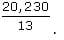We are told that on a randomly chosen day in June the sum of all deposits to that day is a prime integer greater than 100. We are then asked to find the probability that the average daily deposit up to that day contains fewer than 5 decimal places.
In order to answer this question, we need to consider how the numerator (the sum of all deposits, which is defined as a prime integer greater than 100) interacts with the denominator (a randomly selected date in June, which must therefore be some number between 1 and 30).
First, are there certain denominators that – no matter the numerator – will always yield a quotient that contains fewer than 5 decimals?
Yes. A fraction composed of any integer numerator and a denominator of 1 will always yield a quotient that contains fewer than 5 decimal places. This takes care of June 1.
In addition, a fraction composed of any integer numerator and a denominator whose prime factorization contains only 2s and/or 5s will always yield a quotient that contains fewer than 5 decimal places. This takes cares of June 2, 4, 5, 8, 10, 16, 20, and 25.
Why does this work? Consider the chart below: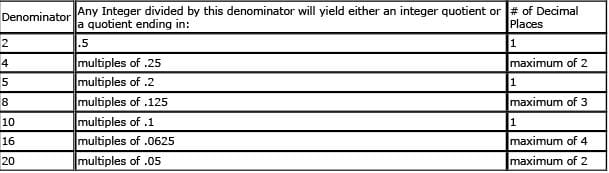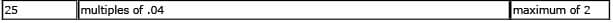What about the other dates in June?
If the chosen day is any other date, the denominator (of the fraction that makes up the average daily deposit) will contain prime factors other than 2 and/or 5 (such as 3 or 7). Recall that the numerator (of the fraction that makes up the average daily deposit) is defined as a prime integer greater than 100 (such as 101).
Thus, the denominator will be composed of at least one prime factor (other than 2 and/or 5) that is not a factor of the numerator. Therefore, when the division takes place, it will result in an infinite decimal. (To understand this principle in greater detail read the explanatory note that follows this solution.) Therefore, of the 30 days in June, only 9 (June 1, 2, 4, 5, 8, 10, 16, 20, and 25) will produce an average daily
deposit that contains fewer than 5 decimal places: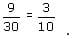Explanatory Note: Why will an infinite decimal result whenever a numerator is divided by a denominator composed of prime factors (other than 2 and/or 5) that are not factors of the numerator?
Consider division as a process that ends when a remainder of 0 is reached.
Let's look at 1 (the numerator) divided by 7 (the denominator), for example. If you divide 1 by 7 on your calculator, you will see that it equals .1428... This decimal will go on infinitely because 7 will never divide evenly into the remainder. That is, a remainder of 0 will never be reached.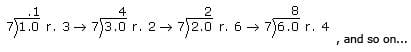For contrast, let's look at 23 divided by 5: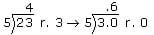So 23/5 = 4.6. When the first remainder is divided by 5, the division will end because the first remainder (3) is treated as if it were a multiple of 10 to facilitate the division and 5 divides evenly into multiples of 10.
By the same token, the remainder when an odd number is divided by 2 is always 1, which is treated as if it were 10 to facilitate the division. 10 divided by 2 is 5 (hence the .5) with no remainder.
When dividing by primes that are not factors of 10 (e.g., 3, 7, 11, etc.), however, the process continues infinitely because the remainders will always be treated as if they were multiples of 10 but the primes cannot divide cleanly into 10, thus creating an endless series of remainders to be divided.
If the divisor contains 2's and/or 5's in addition to other prime factors, the infinite decimal created by the other prime factors will be divided by the 2's and/or 5's but will still be infinite.

Test: Word Problems - 2 - Question 11

A certain cube is composed of 1000 smaller cubes, arranged 10 by 10 by 10. The top layer of cubes is removed from a face, then from the adjacent face above it, then from the adjacent face to the right of the first. The process is repeated on the same three faces in reverse order. Finally, a last layer is taken from the first face. How many smaller cubes have been removed from the larger cube?

Detailed Solution for Test: Word Problems - 2 - Question 11

We can answer this by keeping track of how many cubes are lopped off of each side as the cube is trimmed (10 x 10 + 10 x 9 + 9 x 9 + ...), but this approach is tedious and error prone. A more efficient method is to determine the final dimensions of the trimmed cube, then find the difference between the dimensions of the trimmed and original cubes.
Let's call the first face A, second face B, and third face C. By the end of the operation, we will have removed 2 layers each from faces B and C, and 3 layers from face A. So B now is 8 cubes long, C is 8 cubes long, and A is 7 cubes long. The resulting solid has dimensions 8 x 8 x 7 cubes or 448 cubes.
We began with 1000 cubes, so 1000 - 448 = 552. Thus, 552 cubes have been removed.

Test: Word Problems - 2 - Question 12

The vertical position of an object can be approximated at any given time by the function: p(t) = rt – 5t 2 + b, where p(t) is the vertical position in meters, t is the time in seconds, and r and b are constants. After 2 seconds, the position of an object is 41 meters, and after 5 seconds the position is 26 meters. What is the position of the object, in meters, after 4 seconds?

Detailed Solution for Test: Word Problems - 2 - Question 12

The question gives a function with two unknown constants and two data points. In order to solve for the position of the object after 4 seconds, we need to first solve for the contant s r and b . We can do this by creating two equations from the two data points given: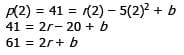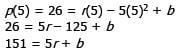We can now solve these equations for r and b using substitution: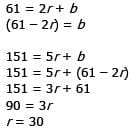Substituting back in, we can find b: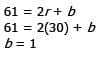So, we can rewrite the original function and plug in t = 4 to find our answer: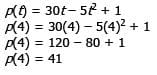Test: Word Problems - 2 - Question 13

A Trussian's weight, in keils, can be calculated by taking the square root of his age in years. A Trussian teenager now weighs three keils less than he will seventeen years after he is twice as old as he is now. How old is he now?

Detailed Solution for Test: Word Problems - 2 - Question 13

Let us call the Trussian's current age a. Therefore the Trussian's current weight is √a Seventeen years after he is twice as old as he is now, the Trussian's age will be 2a+17 and his weight will therefore be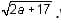We are told that the Trussian's current weight, √a is three keils less than his future weight,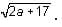Therefore,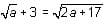We can solve the equation as follows: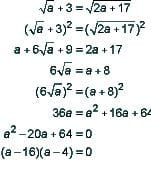a = 16 or 4. However, we are told that the Trussian is a teenager so he must be 16 years old.

Test: Word Problems - 2 - Question 14

Jim went to the bakery to buy donuts for his office mates. He chose a quantity of similar donuts, for which he was charged a total of \$15. As the donuts were being boxed, Jim noticed that a few of them were slightly  ragged-looking so he complained to the clerk. The clerk immediately apologized and then gave Jim 3 extra donuts for free to make up for the damaged goods. As Jim left the shop, he realized that due to the addition of the 3 free donuts, the effective price of the donuts was reduced by \$2 per dozen. How many donuts did Jim receive in the end?

Detailed Solution for Test: Word Problems - 2 - Question 14

Use algebra to solve this problem as follows: Let the x = the number of donuts Jim originally ordered. Since he paid \$15 for these donuts, the price per donut for his original order is \$15/x.
When he leaves, Jim receives 3 free donuts changing the price per donut to \$15/(x + 3). In addition, we know that the price per dozen donuts was \$2 per dozen cheaper when he leaves, equivalent to a per donut savings of \$2/12 = 1/6 dollars. Using this information, we can set up an equation that states that the original price per donut less 1/6 of a dollar is equal to the price per donut after the addition of 3 donuts: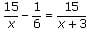We can now solve for x as follows: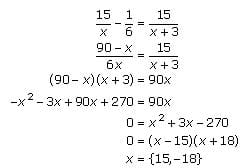The only positive solution of x is 15. Hence, Jim left the donut shop with x + 3 = 18 donuts.

Test: Word Problems - 2 - Question 15

Roberto has three children: two girls and a boy. All were born on the same date in different years. The sum of the ages of the two girls today is smaller than the age of the boy today, but a year from now the sum of the ages of the girls will equal the age of the boy. Three years from today, the difference between the age of the boy and the combined ages of the girls will be

Detailed Solution for Test: Word Problems - 2 - Question 15

Each year, the age of the boy increases by 1. Each year, the sum of the ages of the two girls increases by 2 (as each girl gets older by one year, and there are two of them).
Let's say that the age of the boy today is equal to x, while the combined ages of the girls today is equal to y.
Then, next year the figures will be x + 1 and y + 2, respectively. The problem states that these two figures will be equal, which yields the following equation: x + 1 = y + 2 which can be simplified to x = y + 1 (This is consistent with the fact that the sum of the ages of the two girls today is smaller than the age of the boy today.) Three years from now, the combined age of the girls will be y + 3(2) = y + 6. Three years from now, the boy's age will be x + 3. Using the fact (from above) that x = y + 1, the boy's age three years from now can be written as x + 3 = (y + 1) + 3 = y + 4.
The problem asks for the difference between the age of the boy three years from today and the combined ages of the girls three years from today. This difference equals y + 4 – (y + 6) = –2.